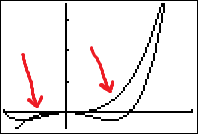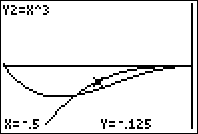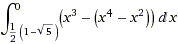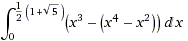# Calculus Problem of the Week September 23, 2011

Sometimes easy and sometimes hard, our calculus problem of the week could come from any calculus topic. If you really want to get better at calculus, following these problems is a great way to make yourself practice! Past calculus problems of the week.

This week’s problem:
(click “see the solution” at the bottom of post to, well, see the solution. If it doesn’t work, please click the title of this post and then try (I’m working on this!))

Find the are enclosed by the graphs of$y=x^4-x^2$ and$y=x^3$.
.

See the solution.

Before you can find the area between the two graphs, you must find where the graphs intersect. These points will be on the boundaries of any region or regions enclosed by the graphs. In order to find where they intersect, you must find where they share the same points – in other words, you must set them equal to each other and solve for x.$x^4-x^2=x^3$$x^4-x^3-x^2=0$$x^2(x^2-x-1)=0$$x^2=0$ and$x^2-x-1=0$

At this stage we have two equations to solve. The first equation is simple and gives us$x=0$. But, the second equation isn’t so simple because it doesn’t factor! In order to solve this, you must use the quadratic formula.$x^2-x-1=0$$x=\dfrac{-(-1)\pm \sqrt{(-1)^2-4(1)(-1)}}{2(1)}$$=\dfrac{1\pm \sqrt{5}}{2}$

We have found that there are three points where the graphs intersect:$x=0$,$x=\dfrac{1+ \sqrt{5}}{2}$, and$=\dfrac{1 - \sqrt{5}}{2}$. The second piece of information we need before we can go ahead and find the area enclosed by the two graphs is which function is “larger” in each enclosed region. Really what I’m looking for is which function is higher on the y-axis than the other. By far, the best method to figure this out is to graph both functions.As you can see above, there are two regions. In the second region, it is clear that$y=x^3$ is the lrager function. But what about the tiny region on the left? Zooming in a bit…Aha!$y=x^3$ is the larger function in both regions. This means the area enclosed by the two graphs in the left hand region is:Also, the area enclosed by the right hand region is:Therefore the total area enclosed by the two graphs is the sum of these two integrals. By far, we have already done the most important part of this. The rest is just calculations and arithmetic and can easily be done by a computer. However, I will show you a little bit of it to show you how far I would go to put this in a calculator. For things like this, doing a little bit of arithmetic before you enter anything into the calculator can make your life much easier.

The first integral:$0 - \left(\dfrac{(\dfrac{1}{2})^4(1-\sqrt(5))^4}{4}-\dfrac{(\dfrac{1}{2})^5(1-\sqrt(5))^5}{5}+\dfrac{(\dfrac{1}{2})^3(1-\sqrt(5))^3}{3}\right) = -\left(\dfrac{(1-\sqrt(5))^4}{64}-\dfrac{(1-\sqrt(5))^5}{160}+\dfrac{(1-\sqrt(5))^3}{24}\right)$

The second integral:$\left(\dfrac{(\dfrac{1}{2})^4(1+\sqrt(5))^4}{4}-\dfrac{(\dfrac{1}{2})^5(1+\sqrt(5))^5}{5}+\dfrac{(\dfrac{1}{2})^3(1+\sqrt(5))^3}{3}\right) - 0 = \dfrac{(1+\sqrt(5))^4}{64}-\dfrac{(1+\sqrt(5))^5}{160}+\dfrac{(1+\sqrt(5))^3}{24}$

Your first integral should give you 0.907514 and the second should give you 0.0241808 for a total area of about 0.931695.

Important note:
If you are like me, you like to check your work and one great way is wolfram alpha. But, there is a bug in the output if you put in “area between y=your function and y=your other function. You can read about the bug here: http://community.wolframalpha.com/viewtopic.php?f=32&t=74585. The better way to check your answer is to type “integral (x^3-(x^4-x^2)) dx” and scroll down. It is able to find both of the integrals we have above so that you can check your work.